This lesson demonstrates that the effects of SPM and anomalous GVD on the pulse propagation are counteracting.

As already discussed, (see the “Group velocity dispersion” in the Tutorials), the effect of GVD on the pulse propagation depends on whether or not the pulse is chirped. With the proper relation between the initial chirp and the GVD parameters, the pulse broadening (which occurs in the absence of any initial chirp) will be preceded by a narrowing stage (pulse compression). On the other hand, the SPM alone leads to pulse chirping, with the sign of the SPM-induced chirp being opposite to that induced by anomalous GVD (see “Effects of SPM” from the Tutorials). This means that in the presence of SPM, the GVD induced pulse-broadening will be reduced (in the case of anomalous), while extra broadening will occur in the case of normal GVD.

Mathematically  the pulse propagation is governed by the nonlinear Schrödinger Equation 1: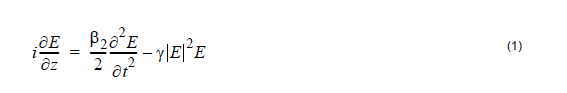The relative importance of GVD and SPM effects depends on the value of the parameter :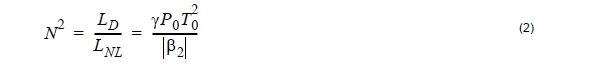where LD and LNL are the dispersion and nonlinear lengths respectively. When
N >> 1, the effects of SPM are dominant and when N < < 1, those of GVD
dominate.

To demonstrate their interplay we choose N=1 and used the simple layout shown in Figure 1. At 40 Gb/s the value of T0 is T0 = 7.5ps.

Figure 1: Layout and parameters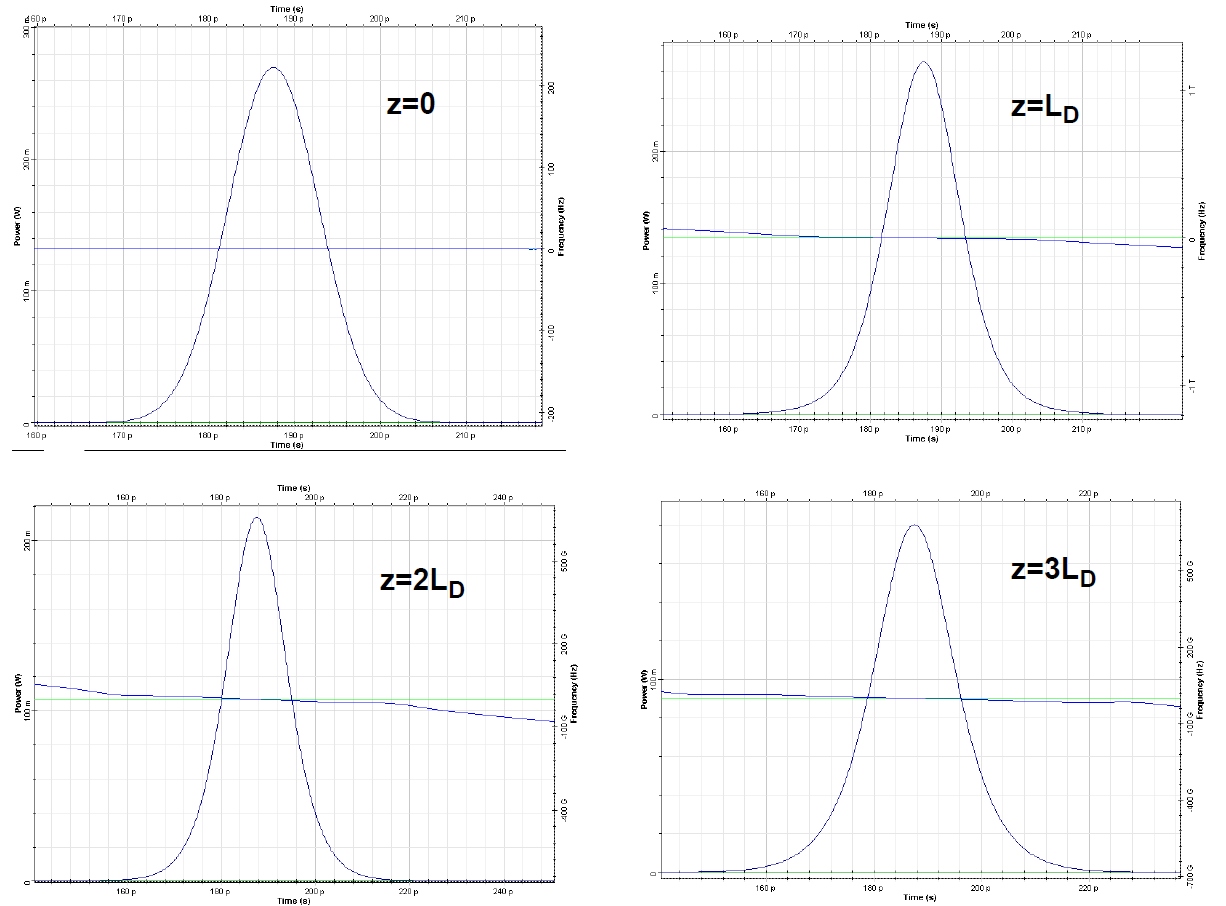Figure 2: Results with SPM

With the default values of: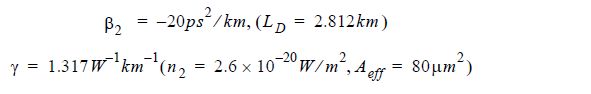in the Nonlinear Dispersive Fiber Total Field component, setting N=1 in Equation 2,
the peak value P0 of the pulse power is P0 = 270mW.

In the Optical Gaussian Pulse Generator, under Main, we enter this value for the
power.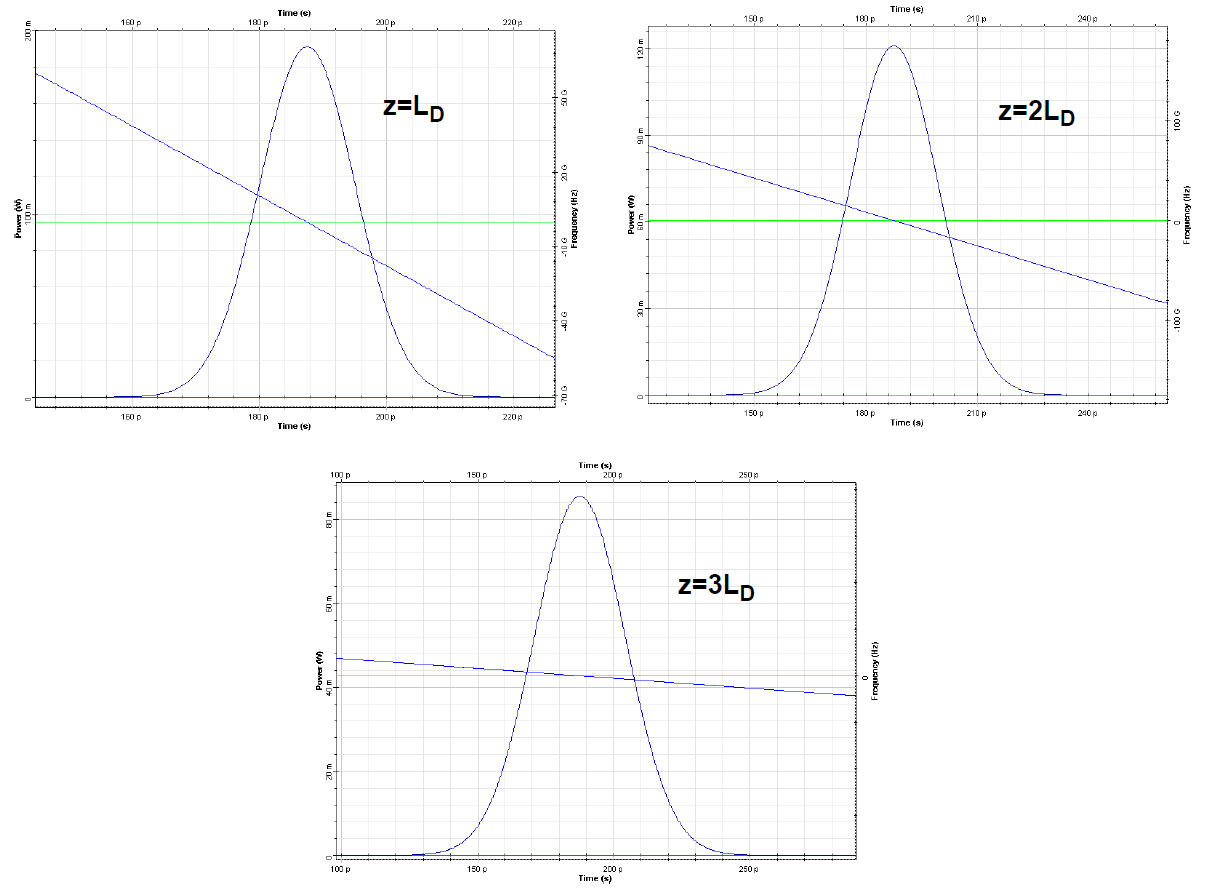Figure 3: Results with disabled SPM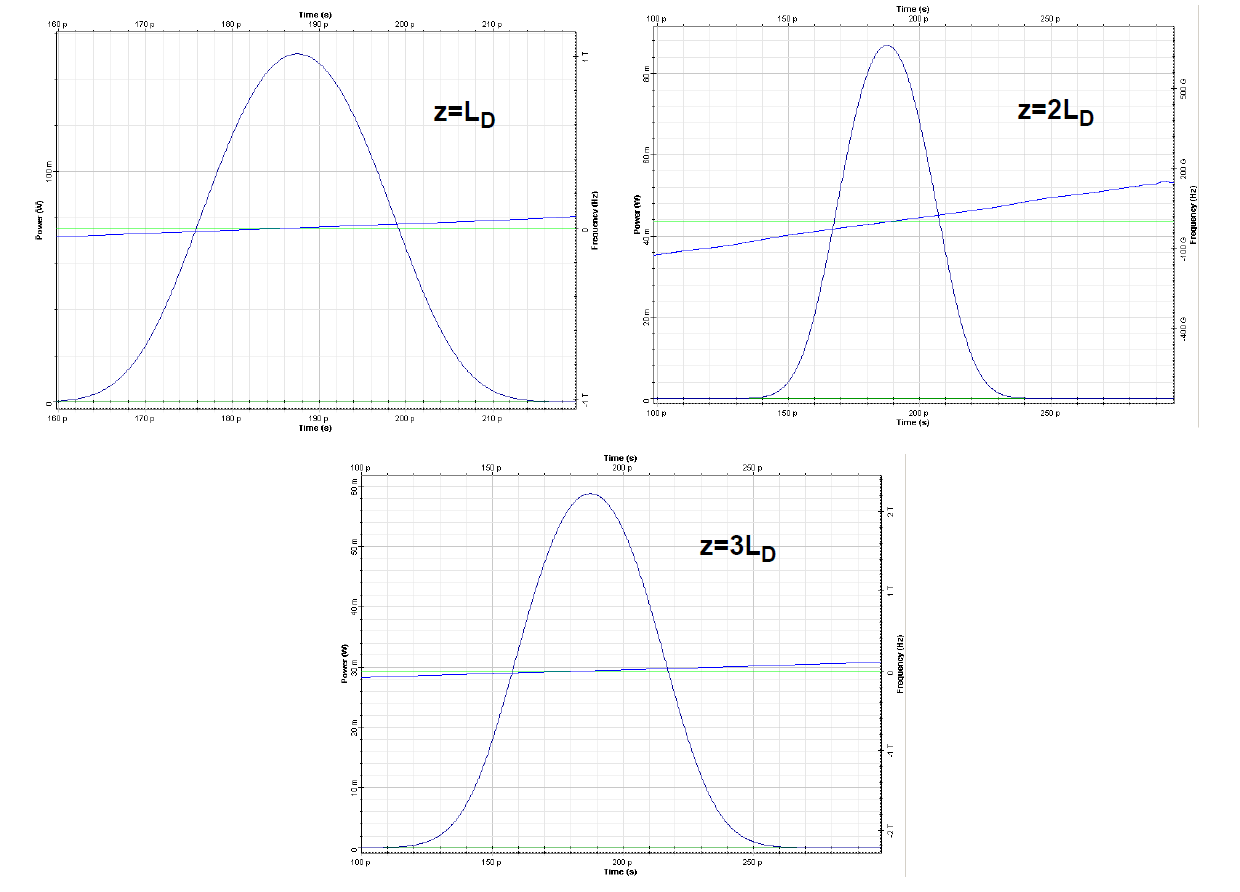Figure 4: Increased pulse broadening and chirp rate

Gaussian pulse propagation in the anomalous GVD regime, taking the SPM into account, is shown in Figure 2. If the effects of SPM are disabled the corresponding results are shown in Figure 3. As one can expect both pulse broadening and pulse chirping are reduced due to the presence of SPM (Figure 2). In this case (anomalous GVD, Figure 2) the blue shifted frequency components travel faster than red-shifted ones. However, SPM generates new frequency components that are red-shifted (slow) near the leading edge and blue-shifted (fast) near the trailing edge (see SPM-induced spectral broadening). This leads to a reduced rate of pulse broadening and chirping. In contrast, in the case of normal GVD, the red-shifted frequency components generated by SPM near the leading edge are faster than the blue shifted components generated at the trailing edge, which leads to an increased pulse broadening and chirping rate, as Figure 4 shows.

Reference:
G. P. Agrawal Nonlinear Fiber Optics, Academic Press (2001).